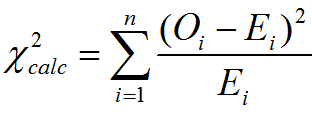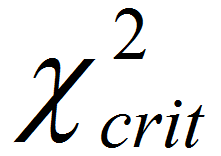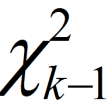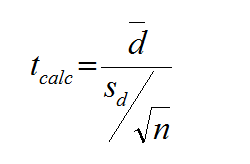# Archive | Analyze Phase## Chi Square Tests with SigmaXL

Chi Square (Contingency Tables) We have looked at hypothesis tests to analyze the proportion of one population vs. a specified value, and the proportions of two populations, but what do we do if we want to analyze more than two populations? A chi-square test is a hypothesis test in which the sampling distribution of the […]## Median Test with SigmaXL

What is Mood’s Median Test? Mood’s median test is a statistical test to compare the medians of two or more populations. The symbol k is the number of groups of our interest and is equal to or greater than two. Mood’s median is an alternative to Kruskal–Wallis. For the data with outliers, Mood’s median test […]## Kruskal Wallis with SigmaXL

Kruskal–Wallis One-Way Analysis of Variance The Kruskal Wallis one-way analysis of variance is a statistical hypothesis test to compare the medians among more than two groups. It is an extension of Mann–Whitney test. While the Mann–Whitney test allows us to compare the samples of two populations, the Kruskal–Wallis test allows us to compare the samples […]## Paired t Test with SigmaXL

Paired t Test The third type of a Two Sample t-Test is the Paired t Test.  This test is used when the two populations are dependent of each other, so each data point from one distribution corresponds to a data point in the other distribution. When using a paired t test, the test statistic is calculated […]